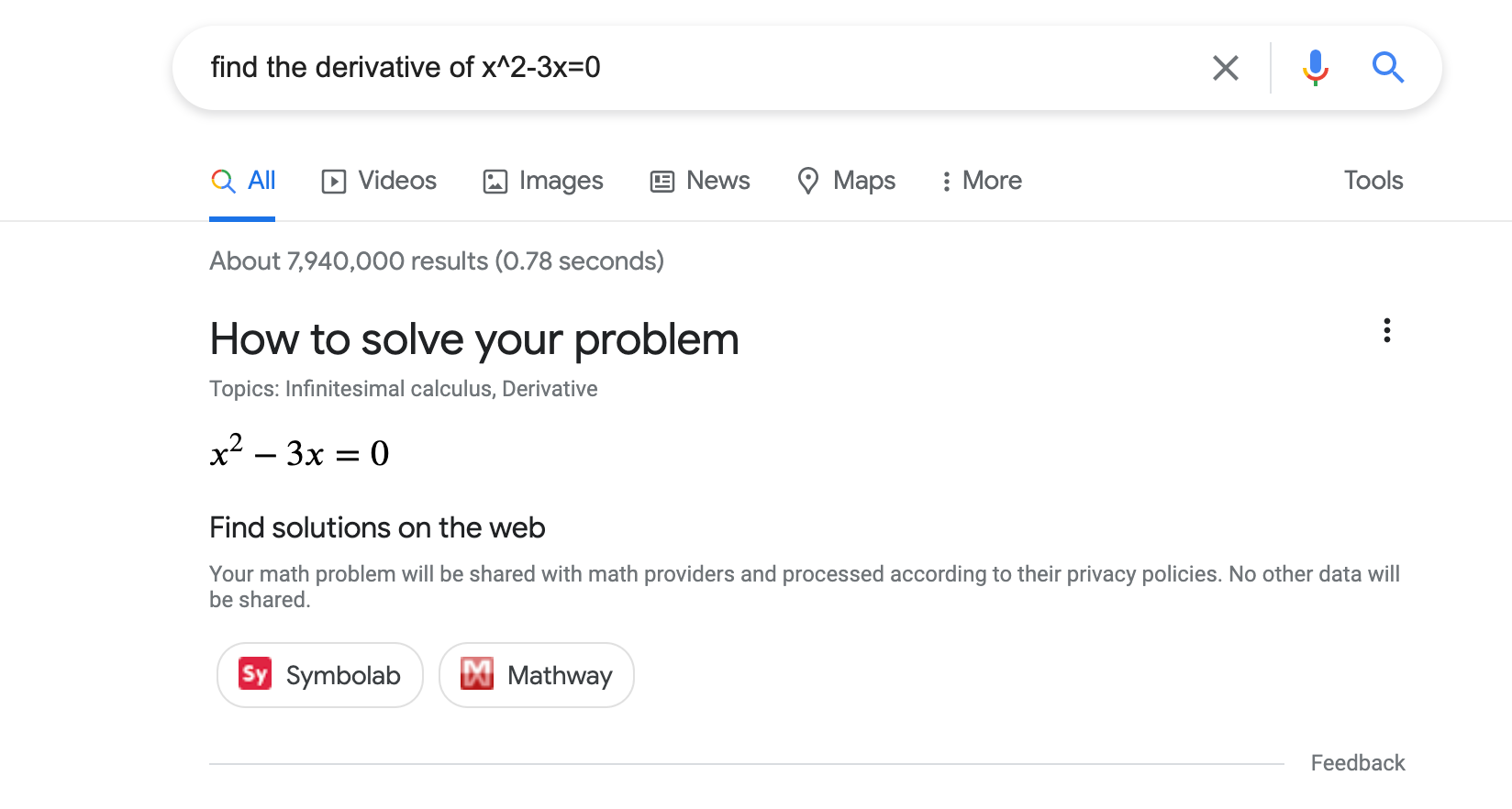# 數學解題工具## 如何新增結構化資料

1. 新增必要屬性。根據您使用的格式，瞭解要在網頁中的什麼位置插入結構化資料
2. 遵循指南規範
3. 使用複合式搜尋結果測試驗證程式碼。
4. 部署幾個包含結構化資料的網頁，並使用網址檢查工具測試 Google 轉譯網頁的情形。請確認 Google 可以存取您的網頁，且網頁並未遭到 robots.txt 檔案或 `noindex` 標記封鎖，也未設有登入規定。如果網頁看起來沒問題，您可以要求 Google 重新檢索您的網址
5. 為了讓 Google 掌握日後的異動內容，建議您提交 Sitemap。您可以使用 Search Console Sitemap API 自動執行這項操作。

## 範例

### 一個解題工具動作

```<html>
<title>An awesome math solver</title>
<body>
<script type="application/ld+json">
{
"@context": "https://schema.org",
"@type": ["MathSolver", "LearningResource"],
"name": "An awesome math solver",
"url": "https://www.mathdomain.com/",
"usageInfo": "https://www.mathdomain.com/privacy",
"inLanguage": "en",
"potentialAction": [{
"@type": "SolveMathAction",
"target": "https://mathdomain.com/solve?q={math_expression_string}",
"mathExpression-input": "required name=math_expression_string",
"eduQuestionType": ["Polynomial Equation","Derivative"]
}],
"learningResourceType": "Math solver"
},
{
"@context": "https://schema.org",
"@type": ["MathSolver", "LearningResource"],
"name": "Un solucionador de matemáticas increíble",
"url": "https://es.mathdomain.com/",
"usageInfo": "https://es.mathdomain.com/privacy",
"inLanguage": "es",
"potentialAction": [{
"@type": "SolveMathAction",
"target": "https://es.mathdomain.com/solve?q={math_expression_string}",
"mathExpression-input": "required name=math_expression_string",
"eduQuestionType": ["Polynomial Equation","Derivative"]
}],
"learningResourceType": "Math solver"
}
</script>
</body>
</html>```

### 兩個解題工具動作

```<html>
<title>An awesome math solver</title>
<body>
<script type="application/ld+json">
{
"@context": "https://schema.org",
"@type": ["MathSolver", "LearningResource"],
"name": "An awesome math solver",
"url": "https://www.mathdomain.com/",
"usageInfo": "https://www.mathdomain.com/privacy",
"inLanguage": "en",
"potentialAction": [{
"@type": "SolveMathAction",
"target": "https://mathdomain.com/solve?q={math_expression_string}",
"mathExpression-input": "required name=math_expression_string",
"eduQuestionType": "Polynomial Equation"
},
{
"@type": "SolveMathAction",
"target": "https://mathdomain.com/trig?q={math_expression_string}",
"mathExpression-input": "required name=math_expression_string",
"eduQuestionType": "Trigonometric Equation"
}],
"learningResourceType": "Math solver"
}
</script>
</body>
</html>```

## 指南規範

### 技術指南

• 請將 `MathSolver` 結構化資料加在網站的首頁。
• 確認您的主機負載設定允許經常進行檢索
• 如果有多個不同網址都包含相同數學解題工具，請在每個網頁的標記中使用標準網址
• 我們嚴禁將數學解題工具完全隱藏在登入機制或付費牆後。當使用者在 Google 上透過這項功能前往您的網站後，必須要能存取原始查詢題目的解題方法和逐步解題說明，其他內容則可以要求登入或採用付費牆。

### 內容指南

• 我們嚴禁偽裝成數學解題工具的宣傳內容，例如由聯盟計畫等第三方張貼的內容。
• 透過這項功能呈現數學解題工具內容時，您有責任確保內容正確無誤且品質優良。如果我們根據品質審查程序，發現您資料中的錯誤達到一定數量，可能會依照嚴重程度移除您的解題工具，直到問題解決。判斷錯誤的條件包括：
• 您的解題工具是否確實能夠解決該類型的問題。
• 解題工具所宣告的解法是否確實能解開該數學問題。

## 結構化資料類型定義

### MathSolver

`MathSolver` 這項工具能提供逐步解法說明，協助學生、教師和其他人解決數學問題。請將 `MathSolver` 結構化資料用於網站首頁。

schema.org/MathSolver 內提供 `MathSolver` 的完整定義。

`potentialAction`

`SolveMathAction`

```
{
"@type": "MathSolver",
"potentialAction": [{
"@type": "SolveMathAction",
"target": "https://mathdomain.com/solve?q={math_expression_string}",
"mathExpression-input": "required name=math_expression_string",
"eduQuestionType": "Polynomial Equation"
}]
}
```
`potentialAction.mathExpression-input`

`Text`

`url`

`URL`

`MathSolver` 的網址。

`usageInfo`

`URL`

```
{
"@type": "MathSolver",
"usageInfo": "https://www.mathdomain.com/privacy"
}```
`potentialAction.target`

`EntryPoint`

```
{
"@type": "MathSolver",
"potentialAction": [{
"@type": "SolveMathAction",
"target": "https://mathdomain.com/solve?q={math_expression_string}"
}]
}```

`inLanguage`

`Text`

```
{
"@type": "MathSolver",
"inLanguage": "es"
}```
`assesses`

`HowTo` 所解決的問題類型。如果您同時使用 `MathSolver` 標記和 `HowTo` 標記，那麼也請使用 `assesses` 屬性。

```
{
"@type": "MathSolver",
"assesses": "Polynomial Equation"
}```
`potentialAction.eduQuestionType`

```
{
"@type": "SolveMathAction",
"eduQuestionType": "Polynomial Equation"
}
```

### LearningResource

`LearningResource` 表示標記主體為一項資源，可以用來協助學生、教師和其他教育學習人士。請將 `LearningResource` 用於網站首頁。

schema.org/LearningResource 內提供 `LearningResource` 的完整定義。

`learningResourceType`

`Text`

```
{
"@type": ["MathSolver", "LearningResource"],
"learningResourceType": "Math Solver"
}```

## 問題類型定義

`Absolute Value Equation`

`Algebra`

`Arc Length`

`Arithmetic`

`Biquadratic Equation`

`Calculus`

`Characteristic Polynomial`

`Circle`

`Derivative`

`Differential Equation`

`Distance`

`Eigenvalue`

`Eigenvector`

`Ellipse`

`Exponential Equation`

`Function`

`Function Composition`

`Geometry`

`Hyperbola`

`Inflection Point`

`Integral`

`Intercept`

`Limit`

`Line Equation`

`Linear Algebra`

`Linear Equation`

`Linear Inequality`

`Logarithmic Equation`

`Logarithmic Inequality`

`Matrix`

{{1,2,5}, {3,-1,1}, {1,2,3}} 簡化列

`Midpoint`

`Parabola`

`Parallel`

`Perpendicular`

`Polynomial Equation`

`Polynomial Expression`

`Polynomial Inequality`

`Quadratic Equation`

`Quadratic Expression`

`Quadratic Inequality`

`Radical Equation`

`Radical Inequality`

`Rational Equation`

`Rational Expression`

`Rational Inequality`

`Slope`

`Statistics`

`System of Equations`

`Trigonometry`

[{ "type": "thumb-down", "id": "missingTheInformationINeed", "label":"缺少我需要的資訊" },{ "type": "thumb-down", "id": "tooComplicatedTooManySteps", "label":"過於複雜/步驟過多" },{ "type": "thumb-down", "id": "outOfDate", "label":"過時" },{ "type": "thumb-down", "id": "translationIssue", "label":"翻譯問題" },{ "type": "thumb-down", "id": "samplesCodeIssue", "label":"示例/程式碼問題" },{ "type": "thumb-down", "id": "otherDown", "label":"其他" }]
[{ "type": "thumb-up", "id": "easyToUnderstand", "label":"容易理解" },{ "type": "thumb-up", "id": "solvedMyProblem", "label":"確實解決了我的問題" },{ "type": "thumb-up", "id": "otherUp", "label":"其他" }]